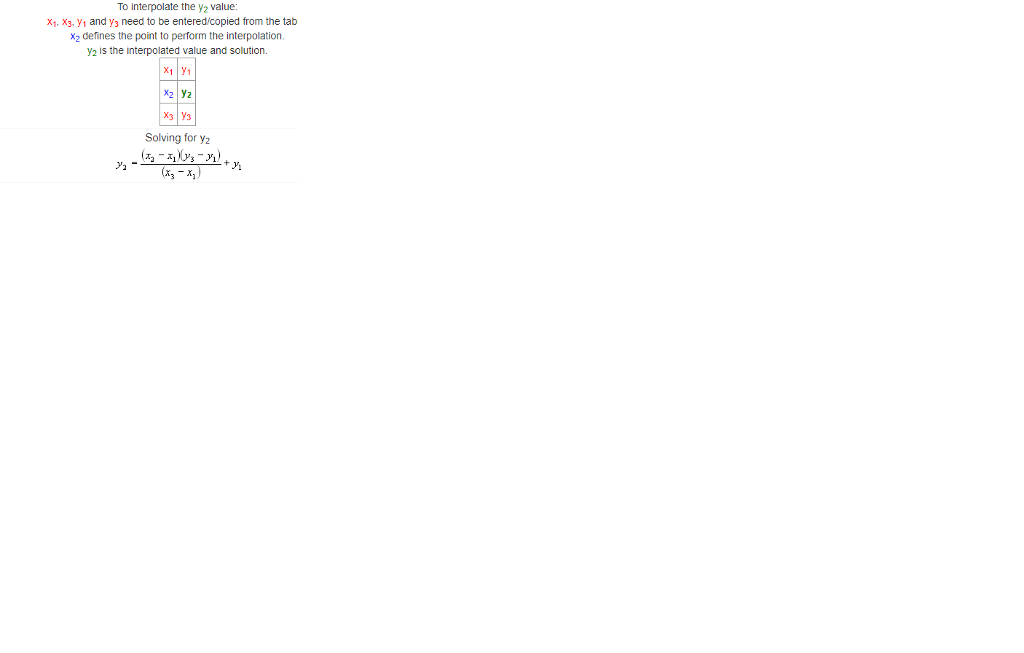# Homework Solution: Linear interpolation is a method of computing the approximate value of a function in one argument, given…

Linear interpolation is a method of computing the approximate value of a function in one argument, given only samples of the function at a set of points. This is commonly used where the values of a function are difficult or expensive to obtain. For example, we may have to carry out a physical experiment, or a time-consuming simulation, to find the function value for a given argument. As an example, think of “deformation of the vehicle passenger compartment in a head-on collision as a function of speed” - to sample the function for a given speed value, we may need do a crash test! Suppose we know the function value at a set of points yi = f(xi), for i = 1…n. To approximate the function at a new point x’, we find the closest known points below and above, say xbelow < x’ and xabove > x’, draw a straight line between (xbelow, ybelow) and (xabove, yabove), and take the value y’ where this line is at x’. The general form of the straight line is a * x + b, where a = (yabove - ybelow) / (xabove - xbelow) and b = ybelow - a * xbelow. Using this, we can calculate y’ = a * x’ + b Task: Your task is to implement a function interpolate(x, y, x_test) that computes the linear interpolation of the unknown function f at a new point x_test. The sample is given in the form of two sequences x and y. Both sequences have the same length, and their elements are numbers. The x sequence contains the points where the function has been sampled, and the y sequence contains the function value at the corresponding point. In other words, y[i] = f(x[i]). Assumptions and restrictions: You can assume that the arguments are as described: that is, x and y are sequences, both have the same length, and their elements are numbers. You should NOT make any assumption about what type of sequence the x and y arguments are. You can assume that the values in x are ordered in increasing order, and that they are unique (that is, there are no repeated x-values). You can assume that x_test is a number, and it is between two values in the x sequence, or possibly equal to a value in the sequence. If x_test is equal to a sample value (a value in the input xsequence), your function should simply return the corresponding function value from y. Your function must return a number. The scipy library has a whole module, scipy.interpolate which performs various kinds of interpolation, including linear interpolation as described above. Obviously, you may not use this module, or any other module that provides a ready-made solution to the problem, since the goal of the assignment is for you to demonstrate that you can implement the function yourself. You can of course use the scipy interpolation function as a reference to test your implementation. NOTE: must be done on python 3, using only function definitions

Rectidirect interpolation is a system of computing the benjoin appraise of a exercise in single evidence, absorbed merely cases of the exercise at a regular of purposes. This is commmerely explanationd where the appraises of a exercise are trying or valuable to conciliate. Control stance, we may accept to propel extinguished a corporeal criterion, or a time-consuming boldness, to ascertain the exercise appraise control a absorbed evidence. As an stance, contemplate of “deformation of the deportment passenger allotment in a head-on clash as a exercise of urge” – to case the exercise control a absorbed urge appraise, we may need do a jar criterion!

Suppose we understand the exercise appraise at a regular of purposes yi = f(xi), control i = 1…n. To benjoin the exercise at a odd purpose x’, we ascertain the closest understandn purposes adown and overhead, say xadown < x’ and xoverhead > x’, sketch a undeviating verse betwixt (xbelow, ybelow) and (xabove, yabove), and transfer the appraise y’ where this verse is at x’.

The open controlm of the undeviating verse is a * x + b, where a = (yoverhead – ybelow) / (xoverhead – xbelow) and b = yadown – a * xbelow. Using this, we can calculate y’ = a * x’ + b

Your character is to tool a exercise interpolate(x, y, x_test) that computes the versear interpolation of the obscure exercise f at a odd purpose x_test. The case is absorbed in the controlm of span posterioritys x and y. Twain posterioritys accept the identical extension, and their elements are aggregate. The x posteriority contains the purposes where the exercise has been cased, and the y posteriority contains the exercise appraise at the identical purpose. In other signification, y[i] = f(x[i]).

Assumptions and restrictions:

You can take that the evidences are as vivid: that is, x and y are posterioritys, twain accept the identical extension, and their elements are aggregate.

You should NOT effect any boldness abextinguished what expression of posteriority the x and y evidences are.

You can take that the appraises in x are enjoined in increasing enjoin, and that they are choice (that is, there are no common x-values).

You can take that x_criterion is a reckon, and it is betwixt span appraises in the x posteriority, or maybe resembling to a appraise in the posteriority. If x_criterion is resembling to a case appraise (a appraise in the input xsequence), your exercise should barely yield the identical exercise appraise from y.

Your exercise must yield a reckon.

The scipy library has a unimpaired module, scipy.import which performs different kinds of interpolation, including versear interpolation as vivid overhead. Obviously, you may not attributable attributable attributable explanation this module, or any other module that provides a ready-made separation to the problem, since the sight of the assignment is control you to evince that you can tool the exercise yourself. You can of plan explanation the scipy interpolation exercise as a intimation to criterion your toolation.

NOTE: must be dsingle on python 3, using merely exercise definitions

## Expert Repartee